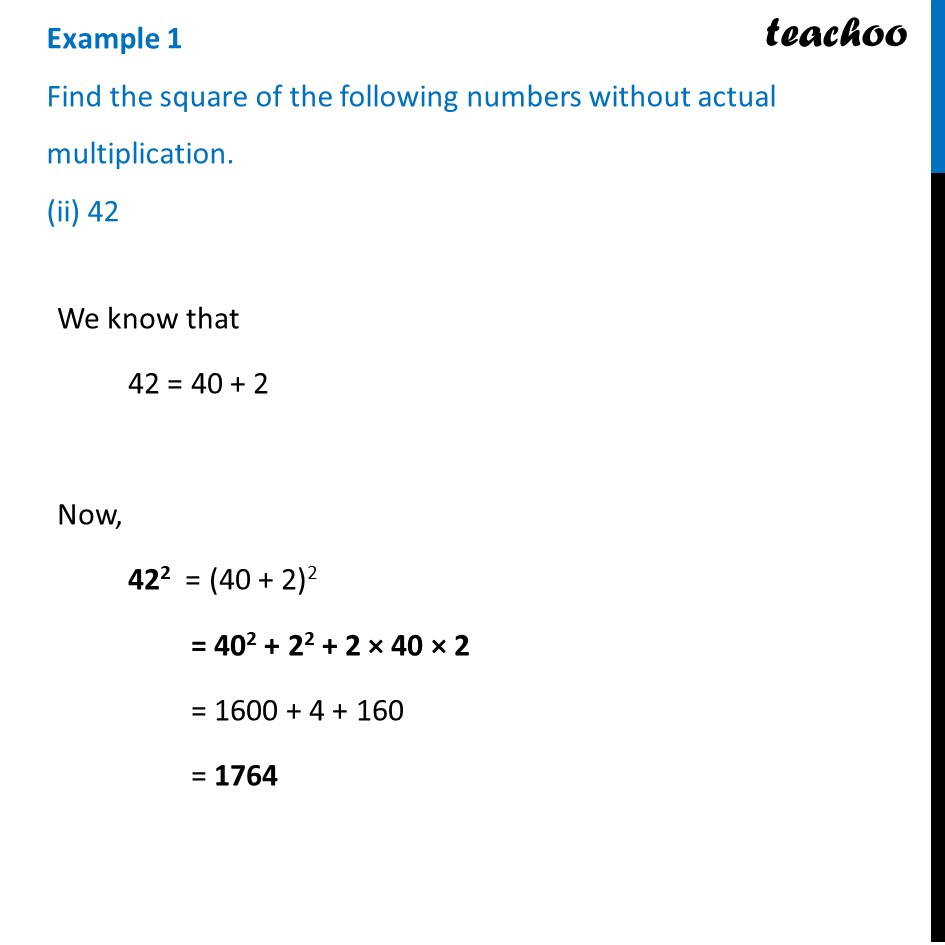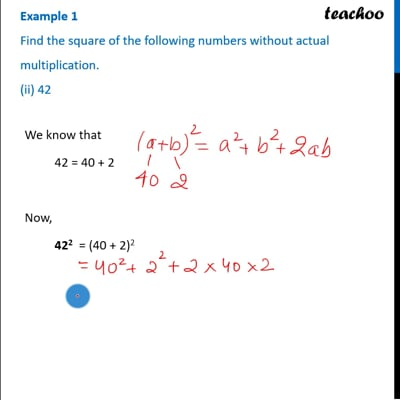Examples

Chapter 6 Class 8 Squares and Square Roots
Serial order wiseThis video is only available for Teachoo black users

Introducing your new favourite teacher - Teachoo Black, at only ₹83 per month

### Transcript

Example 1 Find the square of the following numbers without actual multiplication. (ii) 42 We know that We know that 42 = 40 + 2 Now, 422 = (40 + 2)2 = 402 + 22 + 2 × 40 × 2 = 1600 + 4 + 160 = 1764